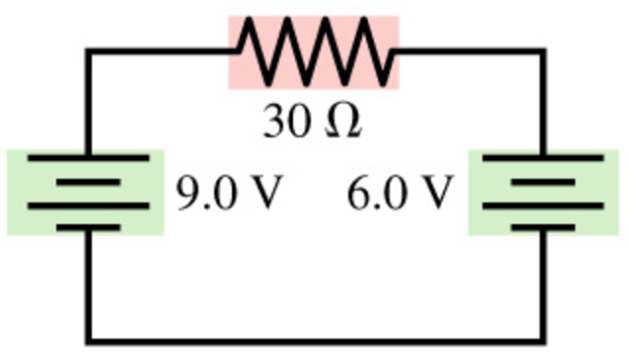# Problem: What is the magnitude of the current in the 30 Ω resistor in the figure (Figure 1)?

###### FREE Expert Solution

Ohm's law:

$\overline{){\mathbf{V}}{\mathbf{=}}{\mathbf{i}}{\mathbf{R}}}$

Solving for current:

Kirchhoff's loop rule:

$\overline{){\mathbf{\Sigma }}{\mathbf{V}}{\mathbf{=}}{\mathbf{0}}}$

6.0 + i(30) - 9.0 = 0

98% (443 ratings)###### Problem Details

What is the magnitude of the current in the 30 Ω resistor in the figure (Figure 1)?